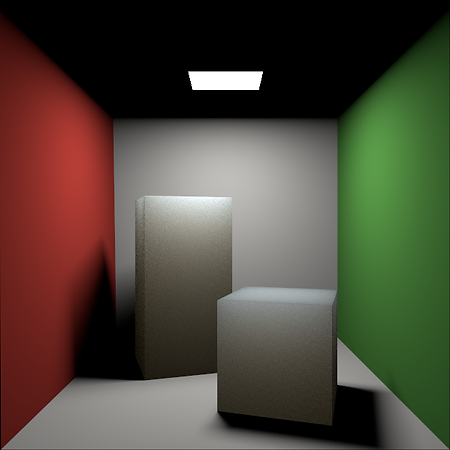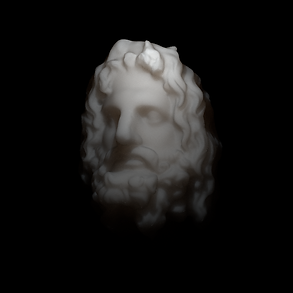###### Subsurface Scattering Implementation in NarumiSubsurface scattering is one of the more complex and interesting material properties to simulate in a renderer. Recently I added subsurface scattering support to Narumi, and I'd like to make a post to discuss the theory and my implementation.

The most common way to model the reflection / scattering property of a material is the familiar BRDF / BSDF, which is a function of a surface position, an incoming direction and an outgoing direction that describes the ratio between the exitant differential radiance and the incident differential irradiance. An important assumption of BSDF is that light always exits from the same position it enters (or at lease the difference is less than pixel footprint). Although this assumption is mostly true for opaque material (such as metal), it does not hold for many organic, translucent objects (such as marble, jade, wax, vegetation, and perhaps more importantly, human skin). To render those objects, we must consider the contribution from light entering other different positions. Thus, BSDF needs to be generalized to BSSRDF that takes a pair of incident/exitant position and a pair of directions to model those objects.

Conceptually, subsurface scattering really is just a subset of the general volumetric scattering problem, formulated from a different perspective. It is totally possible to define the participant media inside a translucent object, and run a volumetric path tracer to render it correctly. The motivation is that compared to ocean water or cloud, the aforementioned objects are "dense", or they are highly scattering. A volumetric path tracer is inefficient at rendering them because we need dozens or even hundreds of ray bounces to simulate the multiple scattering effect. It'd be much better if we can come up with a BSSRDF that implicitly contains the statistical information of multiple scattering (so that we can avoid the expensive bounces). The idea is somewhat similar to the micro-facet model where instead of modeling every facet, we use statistical tools to describe the aggregate properties of all facets.

The classic solution is of course from the 2001 paper of Jensen et al.  where they took inspiration from the classical diffusion theory, and proposed the dipole solution. The rough idea (omitting some details) is as follows:

1. As light travels in a dense, highly scattering medium, it eventually loses all directionality and becomes isotropic after multiple scattering. Thus, the paper simplified the radiance by only taking the 0th and 1st term of its spherical expansion.

2. Use the simplified radiance function and substitute it into the radiance transfer equation, we can obtain the diffusion equation, which is a partial differential equation (PDE) of the 0th term (more formally named fluence rate) and 1st term (vector irradiance). By solving the equation, we will be able to get the result radiance function.

3. Generally solving a PDE requires appropriate boundary condition. Here, a boundary condition means the geometric + illumination setup: what's the shape of the translucent object, and what's the light source. The paper assumes the simplest setup, which is "an infinite plane + an internal point source". The medium is filled below the plane, and air / vacuum is assumed above the plane. It seems weird but the geometry simplification is still reasonable locally (think about how we view earth as a flat plane). The PDE with such boundary condition can then be solved by the dipole method. The dipole method exploits the fact that the PDE has a very simple solution if the setup is "infinite medium (no boundary at all) + a point light", which is called the monopole setup. In order to come up a solution for the infinite plane condition,  we can "superposition" the solutions of a pair of monopoles with each positive and negative radiance (dipole). As a thought experiment, the negative pole is "imaginary", and is used to subtract a portion of the positive pole, so that a plane boundary can be formed. In other words, we can get a complete solution by adding two simple solutions (one positive, and the other negative) together.

4. We now know how to calculate the radiance function due to an internal (point) source below the (infinite planar) surface after multiple scattering. This function only depends on the scattering properties of the material, and thus can be pre-computed once and tabulated. During rendering, we first figure out how much light is transmitted to the surface. We can then directly query the function to get the response after multiple scattering. Finally, we can compute how much light is leaving the boundary.

It's worth noting that the above diffusion-based method does not account for single scattering which has a much stronger directional characteristic. A single scattering term should be added separately. Fortunately, it is simple enough that it can be derived analytically from the radiance transfer equation. And yes, it can also be pre-computed, and stored together with the multiple scattering term.
The BSSRDF derivation is indeed not easy to understand. Besides the original paper, PBRT gave me massive help to understand the theory. I also found Donner's phd thesis  very helpful as it includes a step-by-step derivation with more math details in chapter 5.

The diffusion-based method has been improved gradually. Some of the important improvements include the work of d’Eon and Irving , where they adopted a modified diffusion theory, and the work of Habel et al. , where they proposed photon beam diffusion, which modeled the internal source as a beam which is more accurate than a point. Those improvements has been discussed in  PBRT, and they are not too hard to understand once we understand the original 2001 paper. My implementation also include them.Cornell Box with subsurface scattering material

Above is a rendering of the familiar Cornell Box scene, except that the material of two boxes now has subsurface scattering. The rendering only computes direct lighting for easier visualization, but the translucent look of the material is already obvious. The material uses a scaled version of the scattering parameter set of "wholemilk" measured by Jensen et al. Notice the color shift on the boxes, where the top part exhibits a slight bluish look, while the bottom part exhibits a more red/yellow-ish look. This is because for "wholemilk", the scattering coefficient of blue channel is much larger than that of red channel. After the incoming light (from the ceiling) enters a box, the blue channel of it is more likely to scatter and leave the surface after a short distance. On the other hand, the red channel of the light tend to travel longer in the medium before leaving the surface.Scattering coefficients scaled by 10Scattering coefficients scaled by 25Scattering coefficients scaled by 50Lambertian material (fully opaque)

I also rendered some images of a marble sculpture head, which has more complex geometry and more interesting shape, illuminated by a point light. This time I used the "marble" parameters from Jensen et al. Here are a list of images rendered using the differently scaled, but otherwise same parameters. It can be seen that as the scattering coefficients increase, the appearance becomes more and more opaque. The bottom image is rendered using a simple lambertian material, which can be viewed as a material with infinitely large coefficients where subsurface scattering can be ignored.

It is now also easy to see why simulating subsurface scattering is important. The phenomenon results in various effects such as softened silhouette, subtle color bleeding (even only with direct lighting), and volumetric shadow. These effects all contribute to the realism of the rendering for this kind of materials. By comparison, a simple lambertian model is way too inaccurate and dull-looking.

Finally besides subsurface scattering, I applied a glossy reflection layer to the sculpture, and here is the final rendering. Of course we can then go ahead and add multiple bounces, but I didn't do so here just to save some electricity :)Final sculpture render with scattering coefficients scaled by 50 and glossy reflection

References:

 Jensen, Henrik Wann, et al. "A practical model for subsurface light transport." Proceedings of the 28th annual conference on Computer graphics and interactive techniques. ACM, 2001.

 Donner, Craig Steven. Towards realistic image synthesis of scattering materials. Diss. UC San Diego, 2006.

 d'Eon, Eugene, and Geoffrey Irving. "A quantized-diffusion model for rendering translucent materials." ACM Transactions on Graphics (TOG). Vol. 30. No. 4. ACM, 2011.

 Habel, Ralf, Per H. Christensen, and Wojciech Jarosz. "Photon beam diffusion: A hybrid monte carlo method for subsurface scattering." Computer Graphics Forum. Vol. 32. No. 4. Oxford, UK: Blackwell Publishing Ltd, 2013.